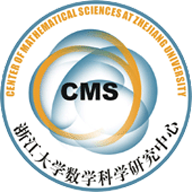(06014) New properties of the intersection numbers on moduli spaces of curves

Abstract. We present certain new properties about the intersection numbers on moduli spaces
of curves $\overline{\sM}_{g,n}$, including a simple explicit formula of $n$-point
functions and several new identities of intersection numbers. In particular we
prove a new identity, which together with a conjectural identity implies the famous
Faber's conjecture about relations in $\mathcal R^{g-2}(\sM_g)$. These new identities
clarify the mysterious constant in Faber's conjecture and uncover novel combinatorial
structures of intersection numbers.

We also discuss some numerical properties of Hodge integrals which have provided
numerous inspirations for this work.

• LiuXuRecursion.pdf